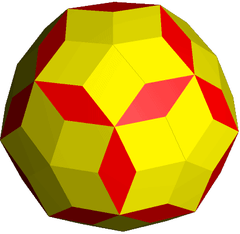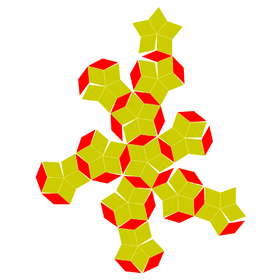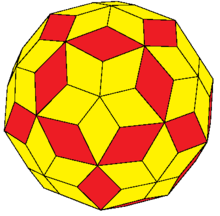# Rhombic enneacontahedron

Rhombic enneacontahedronConway notationjtI = dakD
Typezonohedron
Face polygonrhombus
Faces90 rhombi:
(60 wide and 30 narrow)
Edges180 (60+120)
Vertices92 (12+20+60)
Faces per vertex3, 5, and 6
Dual polyhedronRectified truncated icosahedron
Symmetry groupIh, [5,3], *532
Propertiesconvex, zonohedronNet

A rhombic enneacontahedron (plural: rhombic enneacontahedra) is a polyhedron composed of 90 rhombic faces; with three, five, or six rhombi meeting at each vertex. It has 60 broad rhombi and 30 slim. The rhombic enneacontahedron is a zonohedron with a superficial resemblance to the rhombic triacontahedron.

It can also be seen as a nonuniform truncated icosahedron with pyramids augmented to the pentagonal and hexagonal faces with heights adjusted until the dihedral angles are zero, and the two pyramid type side edges are equal length. This construction is expressed in the Conway polyhedron notation jtI with join operator j. Without the equal edge constraint, the wide rhombi are kites if limited only by the icosahedral symmetry.joined truncated icosahedron

The sixty broad rhombic faces in the rhombic enneacontahedron are identical to those in the rhombic dodecahedron, with diagonals in a ratio of 1 to the square root of 2. The face angles of these rhombi are approximately 70.528° and 109.471°. The thirty slim rhombic faces have face vertex angles of 41.810° and 138.189°; the diagonals are in ratio of 1 to φ2.

It is also called a rhombic enenicontahedron in Lloyd Kahn's Domebook 2.

## Close-packing density

The optimal packing fraction of rhombic enneacontahedra is given by.

It was noticed that this optimal value is obtained in a Bravais lattice by de Graaf (2011). Since the rhombic enneacontahedron is contained in a rhombic dodecahedron whose inscribed sphere is identical to its own inscribed sphere, the value of the optimal packing fraction is a corollary of the Kepler conjecture: it can be achieved by putting a rhombicuboctahedron in each cell of the rhombic dodecahedral honeycomb, and it cannot be surpassed, since otherwise the optimal packing density of spheres could be surpassed by putting a sphere in each rhombicuboctahedron of the hypothetical packing which surpasses it.